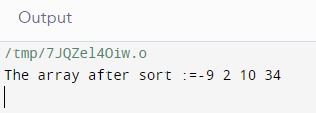### odd-even sort in c++

This algorithm is divided into two phases- Odd and Even Phase. The algorithm runs until the array elements are sorted and in each iteration two phases occurs- Odd and Even Phases.In the odd phase, we perform a bubble sort on odd indexed elements and in the even phase, we perform a bubble sort on even indexed elements.

Sample Program :-

#include <bits/stdc++.h> using namespace std; void oddEvenSort(int arr[], int n) { bool isSorted = false; // Initially array is unsorted while (!isSorted) { isSorted = true; // Perform Bubble sort on odd indexed element for (int i = 1; i <= n - 2; i = i + 2) { if (arr[i] > arr[i + 1]) { swap(arr[i], arr[i + 1]); isSorted = false; } } // Perform Bubble sort on even indexed element for (int i = 0; i <= n - 2; i = i + 2) { if (arr[i] > arr[i + 1]) { swap(arr[i], arr[i + 1]); isSorted = false; } } } return; } // A utility function ot print an array of size n void printArray(int arr[], int n) { for (int i = 0; i < n; i++) cout << arr[i] << " "; cout << "\n"; } // Driver program to test above functions. int main() { int arr[] = { 34, 2, 10, -9 }; int n = sizeof(arr) / sizeof(arr); cout<<"The array after sort :="; oddEvenSort(arr, n); printArray(arr, n); return (0); }

OUTPUT:-